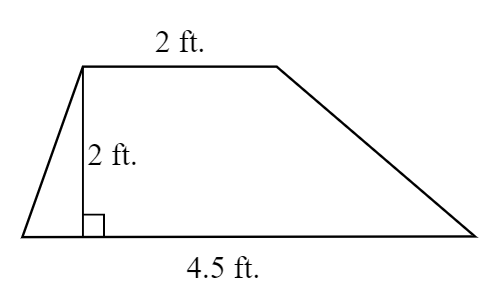The area of a trapezoid is equal to $$[ \frac{\mathrm{(b_1 + b_2)h} }{\mathrm{2} }]$$. For this trapezoid, that is $$\frac{\mathrm{(2+4.5) \times 2} }{\mathrm{2} }$$ = 6.5 square feet.